# RS Aggarwal Solutions for Class 8 Maths Chapter 24 - Pie Charts

RS Aggarwal Solutions for Class 8 Maths Chapter 24- Pie Charts, are provided here. Our expert faculty team has prepared solutions in order to help you with your exam preparation to acquire good marks in Maths. RS Aggarwal Solutions for Class 8 Maths comes in very handy at this point. Our solution module utilizes various shortcut tips and practical examples to explain all the exercise questions in a simple and easily understandable language. Practising the textbook questions will help you to analyze your level of preparation and knowledge of the concept.
In order to help you understand and solve the problems, we at BYJU’S have designed these solutions in a very lucid and clear manner that helps students solve problems in the most efficient possible ways. Here is the RS Aggarwal Solutions for Class 8 Chapter 24 where solutions are solved in detail. Download pdf of Class 8 Chapter 24 in their respective links.

RS Aggarwal Solutions for Class 8 Maths Chapter 24- Pie Charts, are provided here. Our solution module utilizes various shortcut tips and practical examples to explain all the exercise questions in a simple and easily understandable language. Our expert faculty team at BYJU’S has designed these solutions in a very lucid and clear manner that helps students solve problems without any difficulty. Students can easily refer and download pdf of RS Aggarwal Class 8 Maths Solutions book and start practising the textbook questions which will help you in securing high marks in your examination.

## Download PDF of RS Aggarwal Solutions for Class 8 Maths Chapter 24 – Pie Charts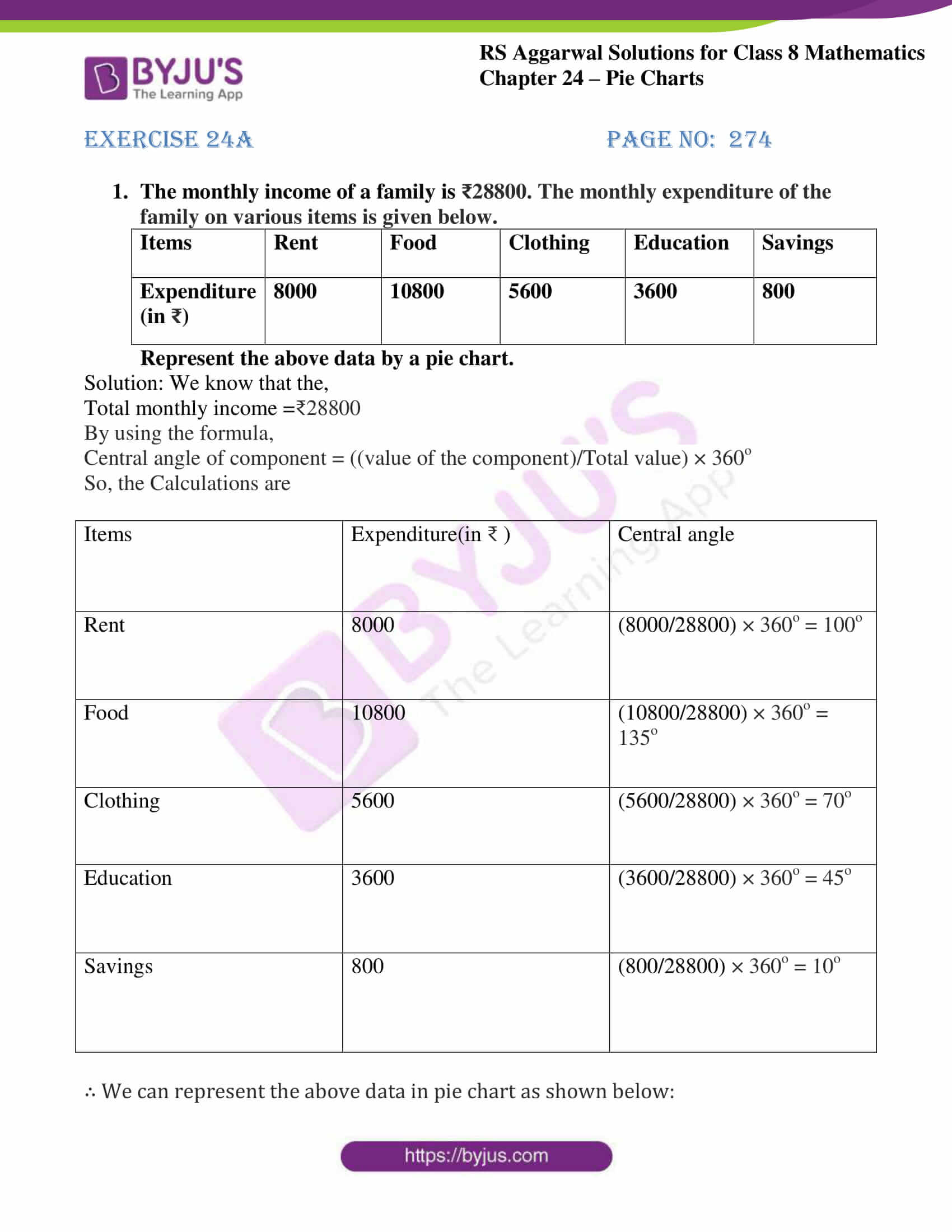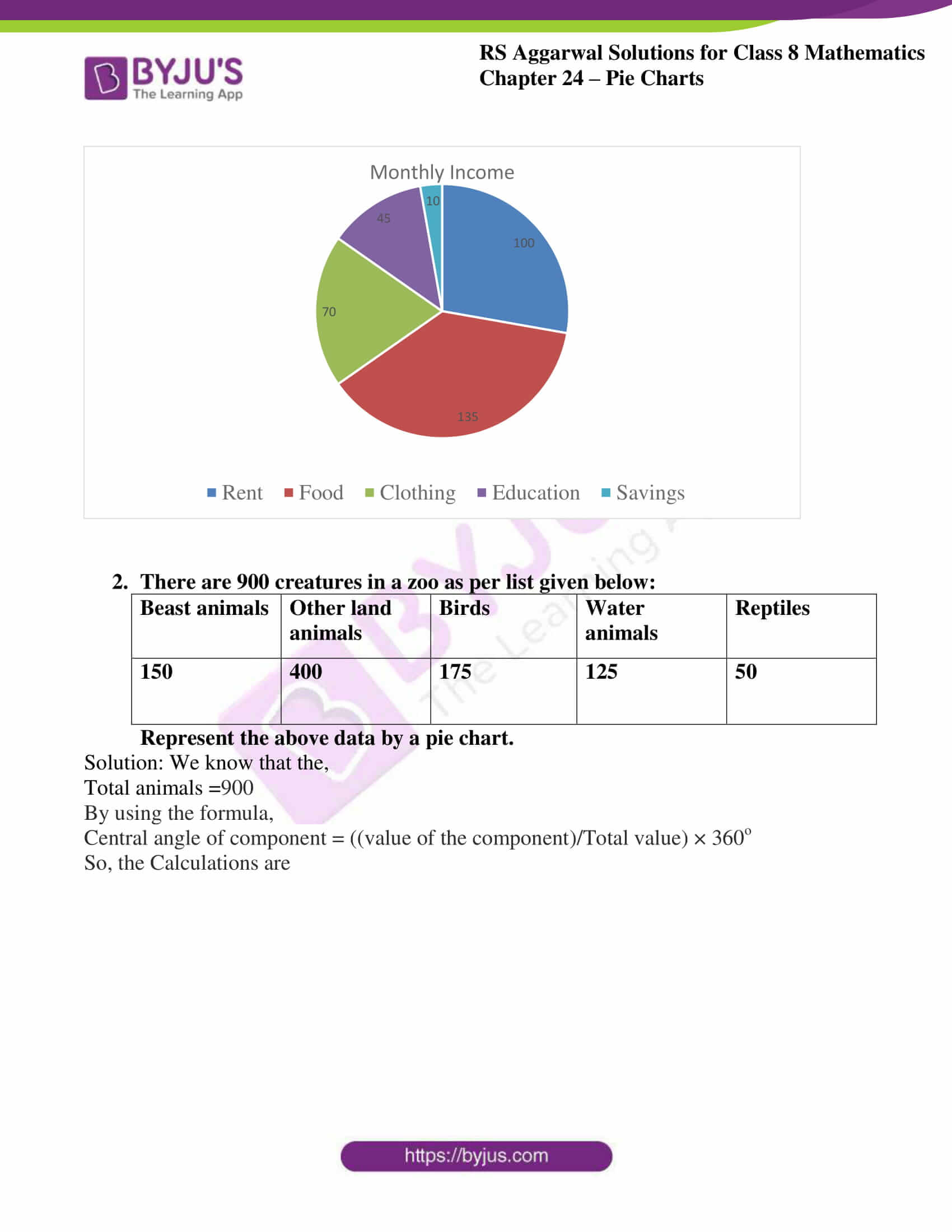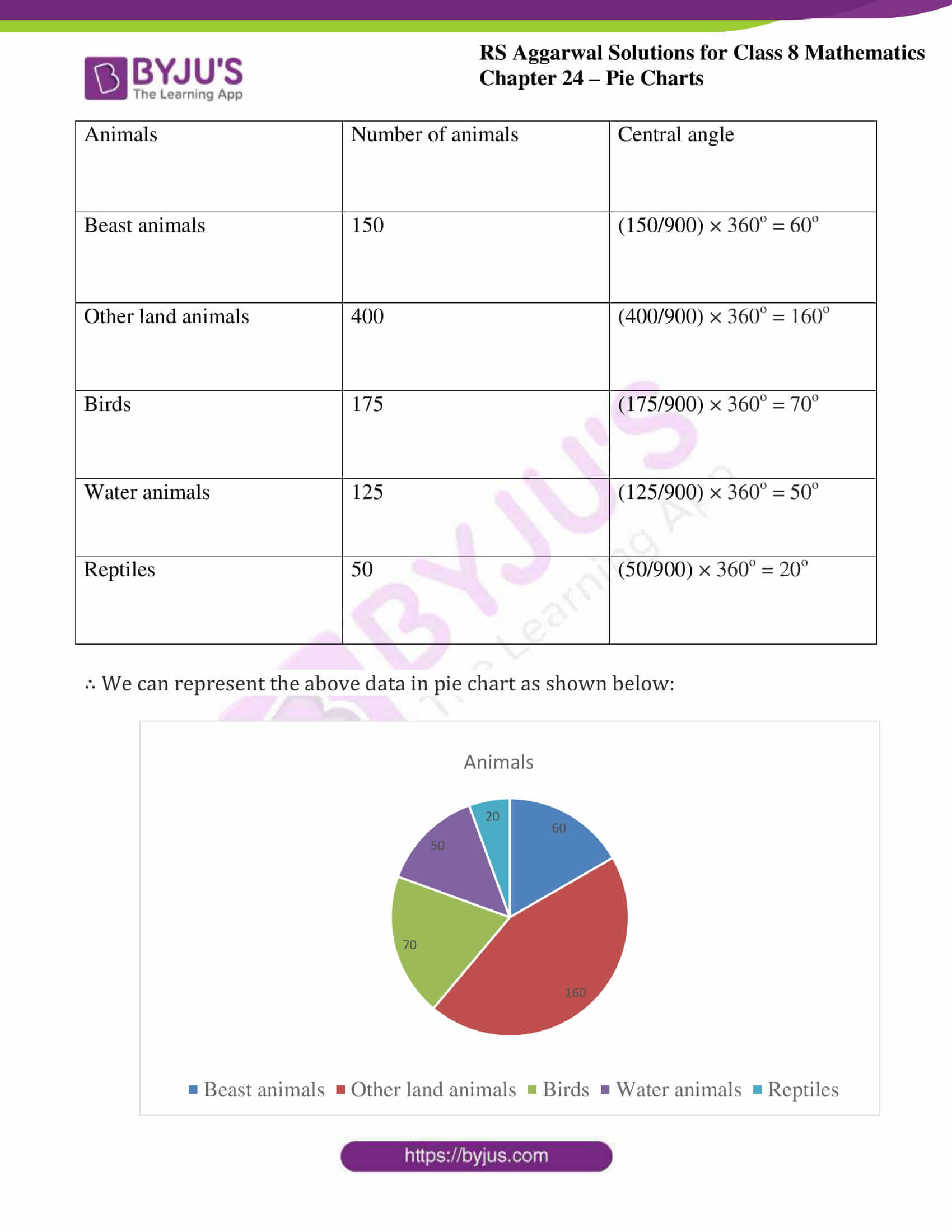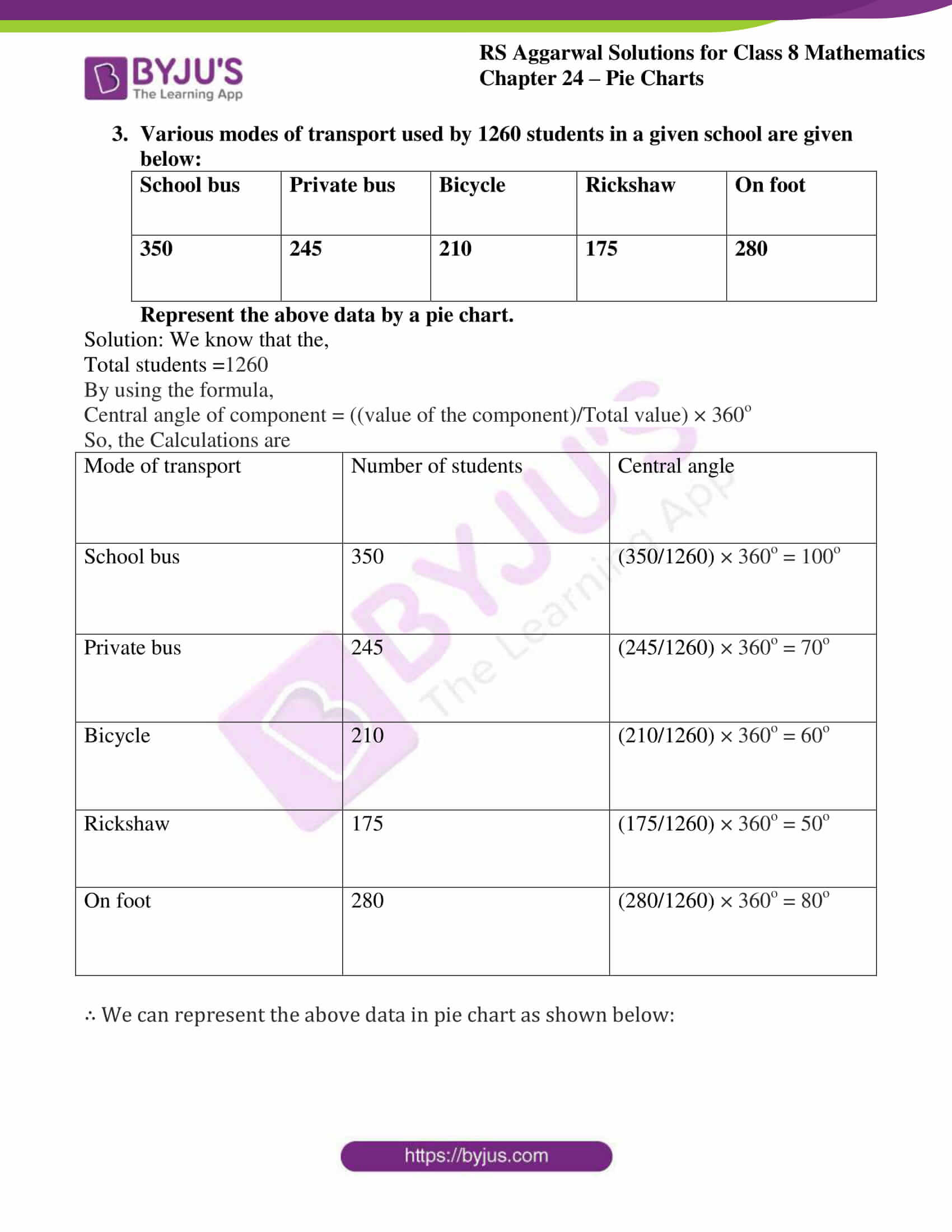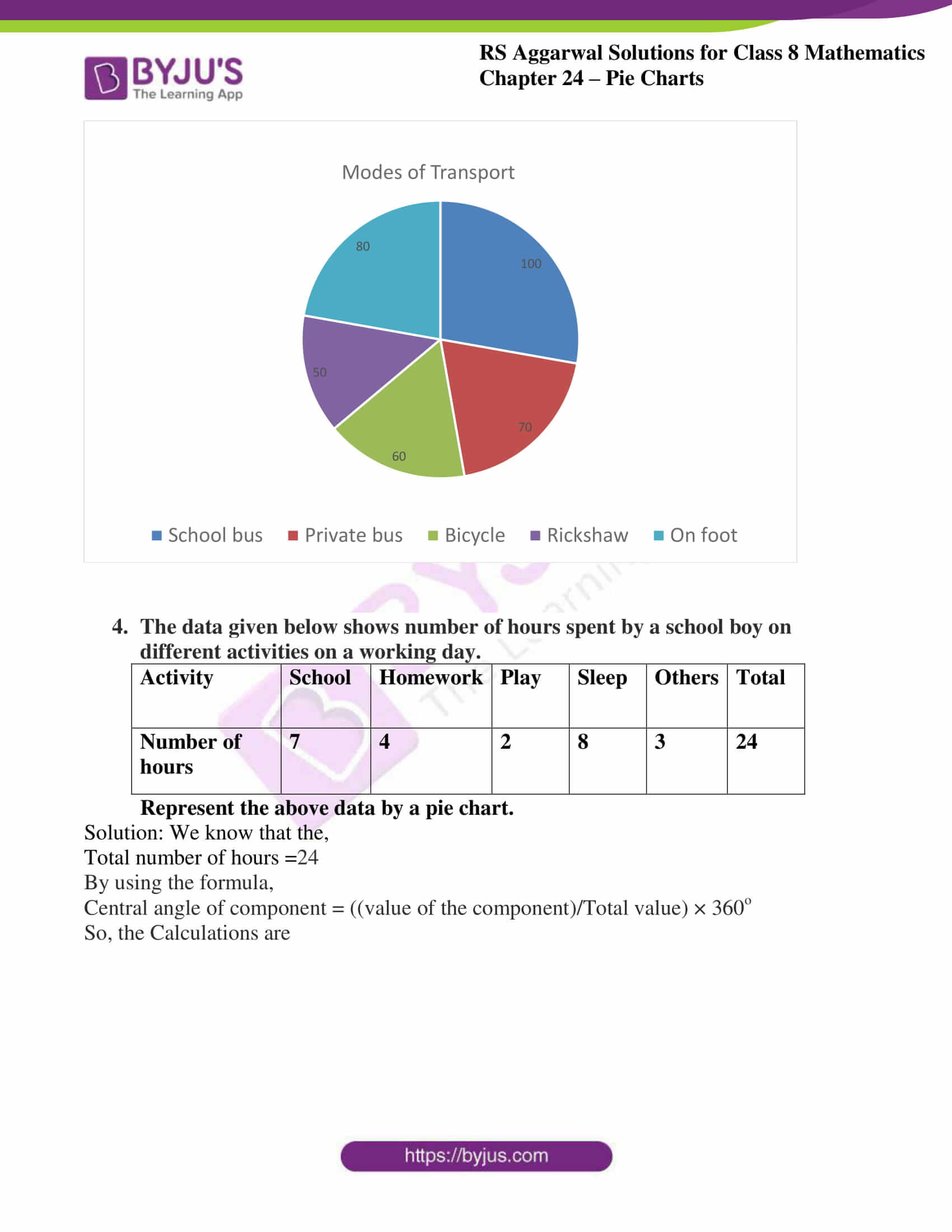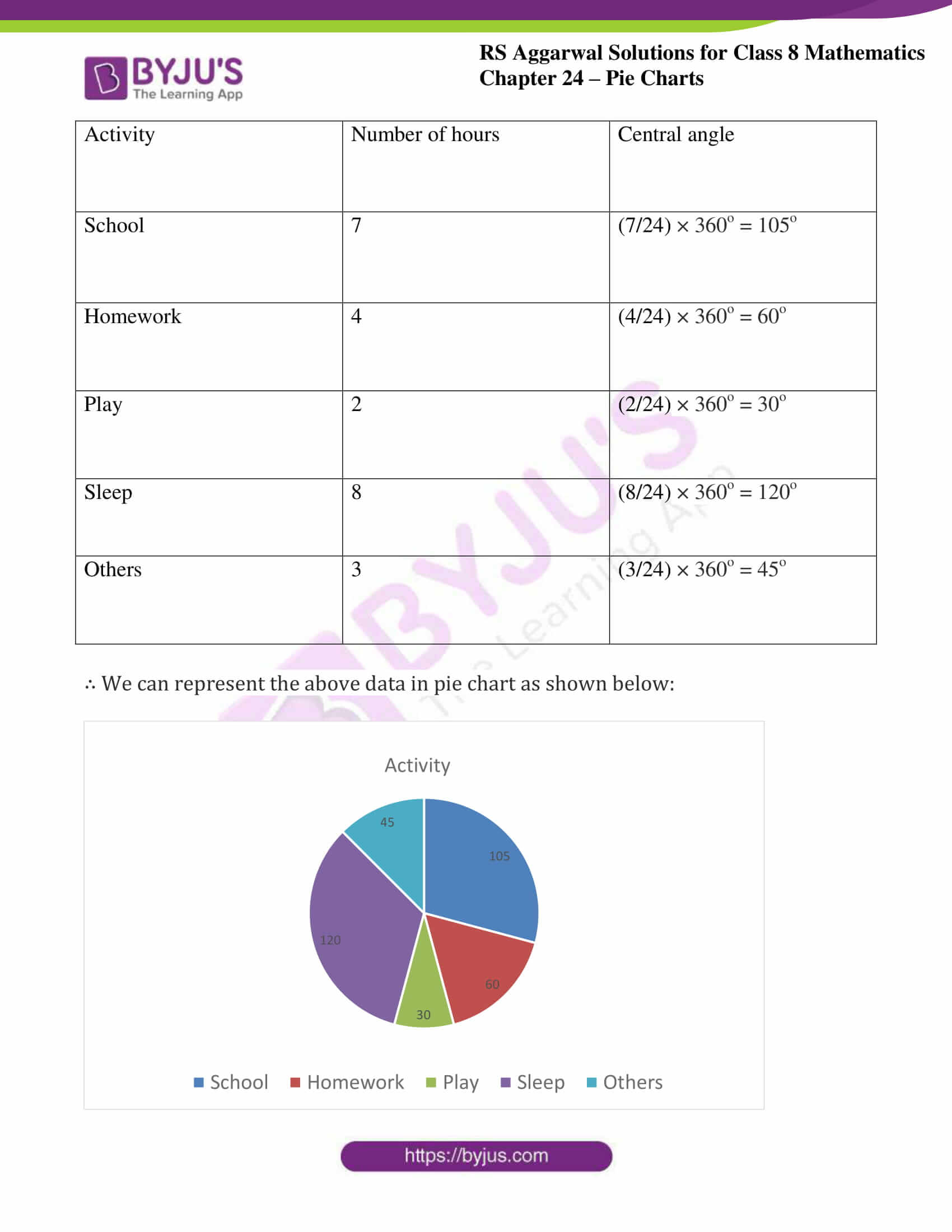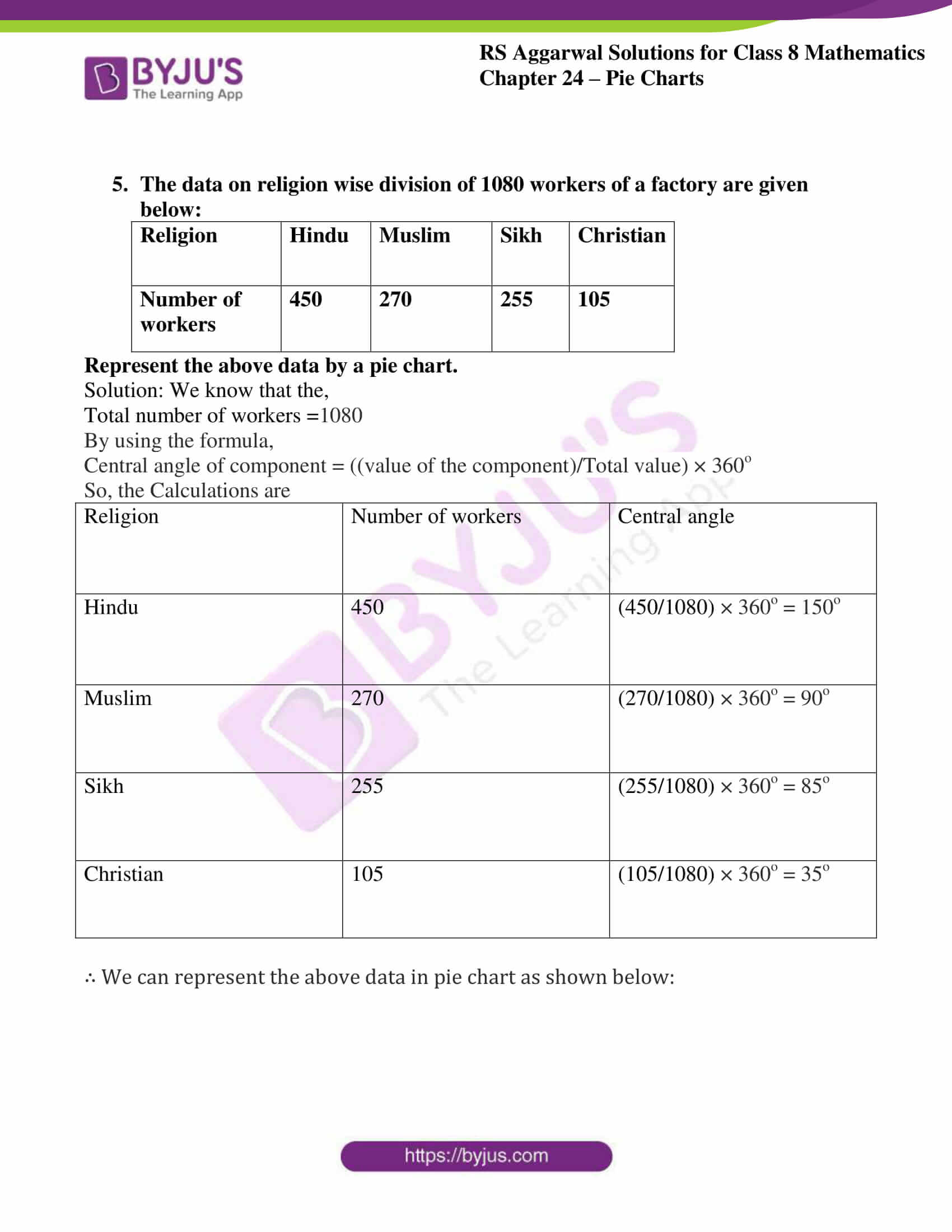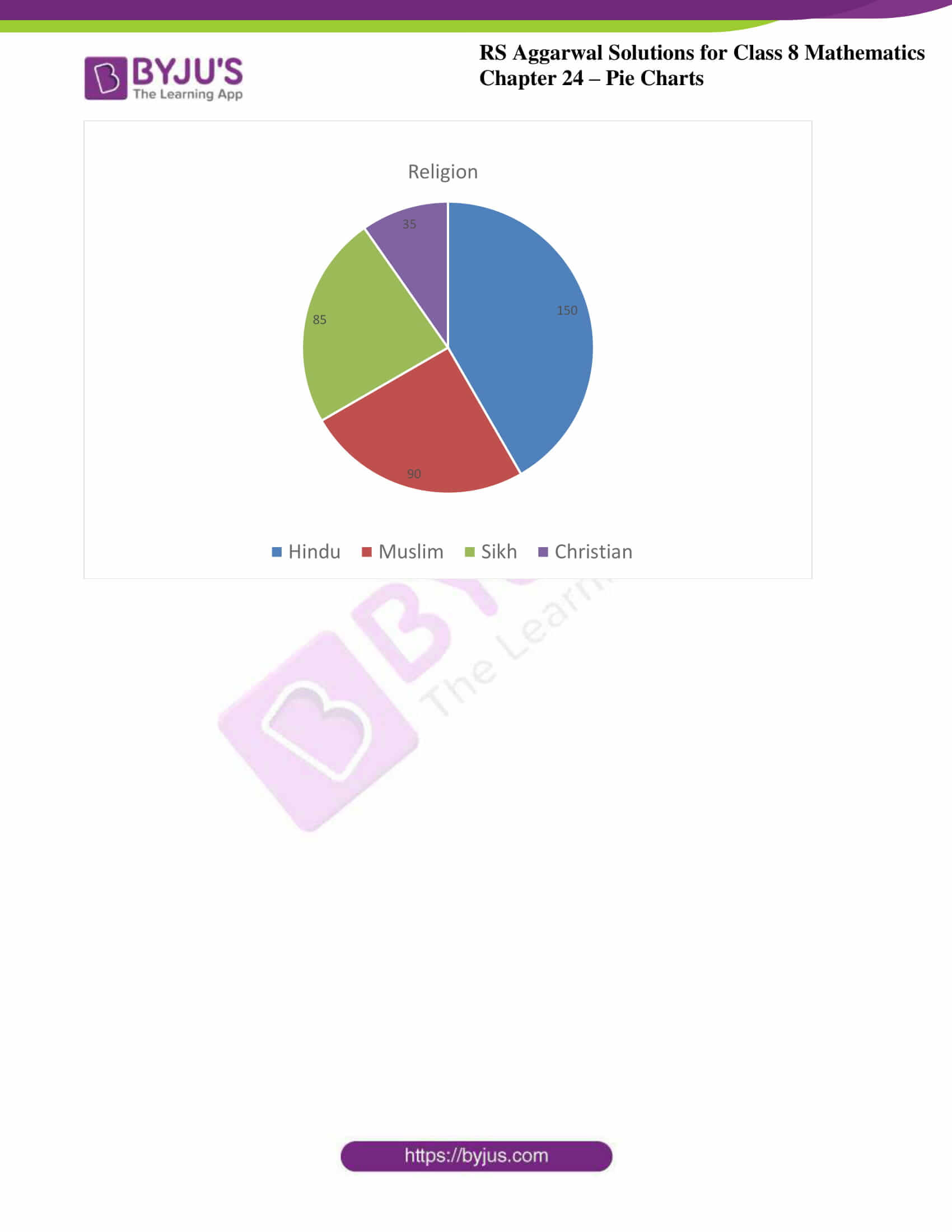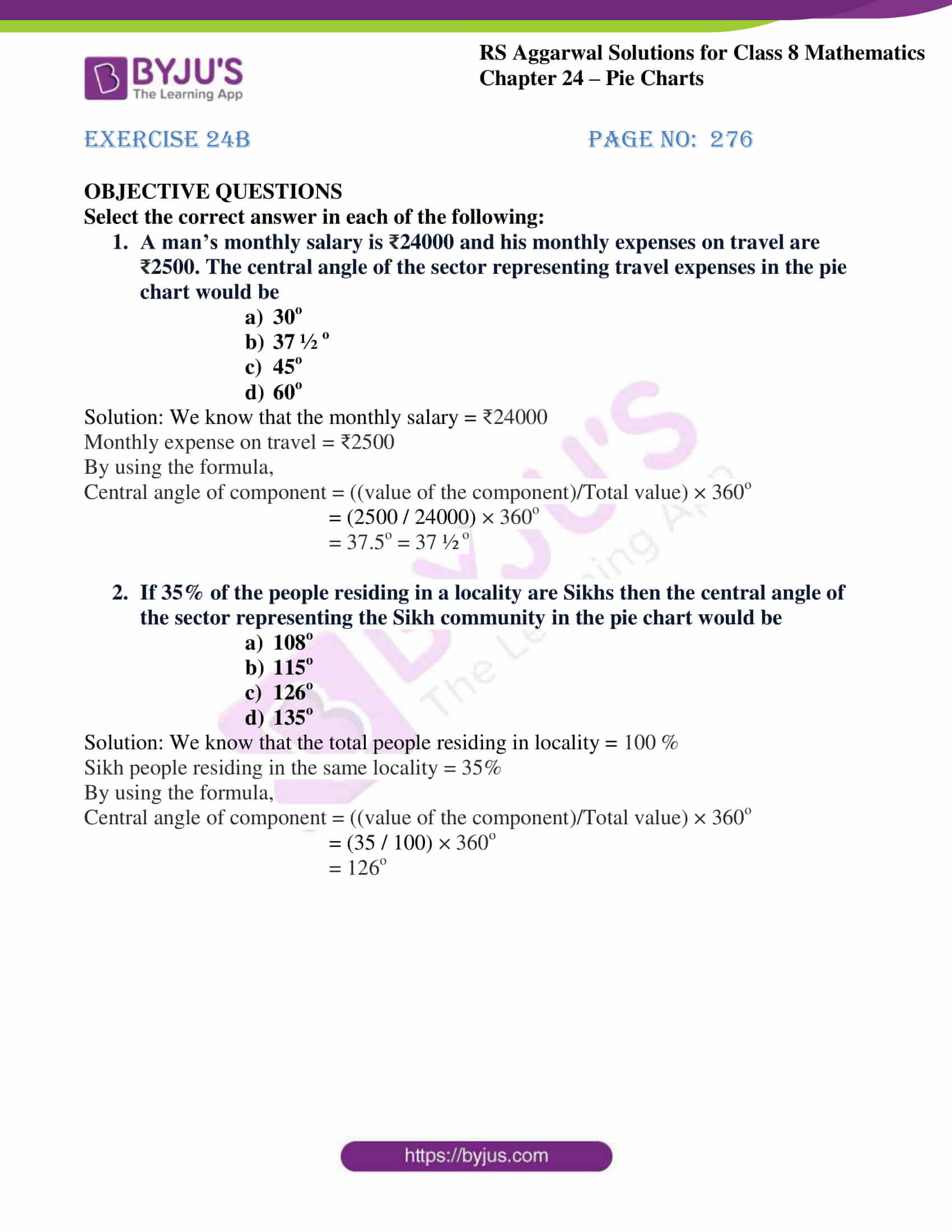## Access answers to RS Aggarwal Solutions for Class 8 Maths Chapter 24 – Pie Charts

Exercise 24A
1. The monthly income of a family is ₹28800. The monthly expenditure of the family on various items is given below.

 Items Rent Food Clothing Education Savings Expenditure (in ₹) 8000 10800 5600 3600 800

Represent the above data by a pie chart.
Solution: We know that the,
Total monthly income = ₹28800
By using the formula,
Central angle of component = ((value of the component)/Total value) × 360o
So, the Calculations are

 Items Expenditure(in ₹ ) Central angle Rent 8000 (8000/28800) × 360o = 100o Food 10800 (10800/28800) × 360o = 135o Clothing 5600 (5600/28800) × 360o = 70o Education 3600 (3600/28800) × 360o = 45o Savings 800 (800/28800) × 360o = 10o

∴ We can represent the above data in pie chart as shown below: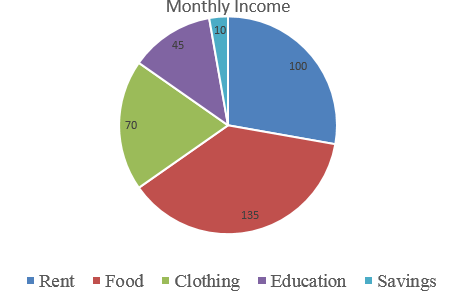2. There are 900 creatures in a zoo as per list given below:

 Beast animals Other land animals Birds Water animals Reptiles 150 400 175 125 50

Represent the above data by a pie chart.
Solution: We know that the,
Total animals =900
By using the formula,
Central angle of component = ((value of the component)/Total value) × 360o
So, the Calculations are

 Animals Number of animals Central angle Beast animals 150 (150/900) × 360o = 60o Other land animals 400 (400/900) × 360o = 160o Birds 175 (175/900) × 360o = 70o Water animals 125 (125/900) × 360o = 50o Reptiles 50 (50/900) × 360o = 20o

∴ We can represent the above data in pie chart as shown below: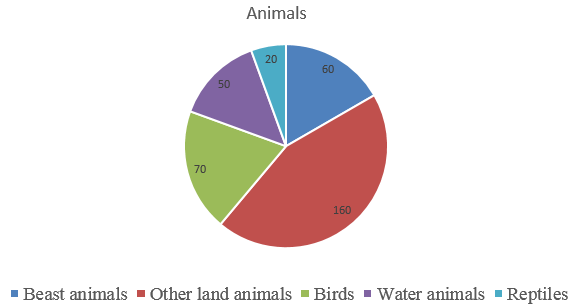3. Various modes of transport used by 1260 students in a given school are given below:

 School bus Private bus Bicycle Rickshaw On foot 350 245 210 175 280

Represent the above data by a pie chart.
Solution: We know that the,
Total students =1260
By using the formula,
Central angle of component = ((value of the component)/Total value) × 360o
So, the Calculations are

 Mode of transport Number of students Central angle School bus 350 (350/1260) × 360o = 100o Private bus 245 (245/1260) × 360o = 70o Bicycle 210 (210/1260) × 360o = 60o Rickshaw 175 (175/1260) × 360o = 50o On foot 280 (280/1260) × 360o = 80o

∴ We can represent the above data in pie chart as shown below: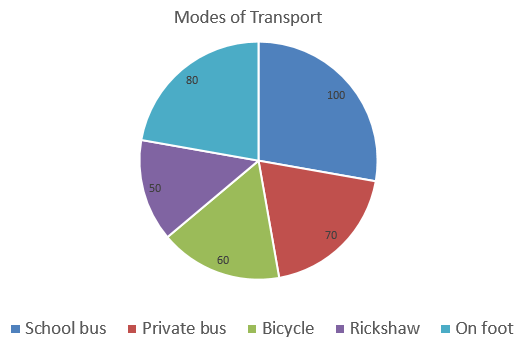4. The data given below shows number of hours spent by a school boy on different activities on a working day.

 Activity School Homework Play Sleep Others Total Number of hours 7 4 2 8 3 24

Represent the above data by a pie chart.
Solution: We know that the,
Total number of hours =24
By using the formula,
Central angle of component = ((value of the component)/Total value) × 360o
So, the Calculations are

 Activity Number of hours Central angle School 7 (7/24) × 360o = 105o Homework 4 (4/24) × 360o = 60o Play 2 (2/24) × 360o = 30o Sleep 8 (8/24) × 360o = 120o Others 3 (3/24) × 360o = 45o

∴ We can represent the above data in pie chart as shown below: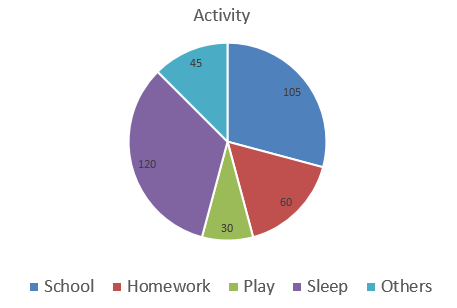5. The data on religion wise division of 1080 workers of a factory are given below:

 Religion Hindu Muslim Sikh Christian Number of workers 450 270 255 105

Represent the above data by a pie chart.
Solution: We know that the,
Total number of workers =1080
By using the formula,
Central angle of component = ((value of the component)/Total value) × 360o
So, the Calculations are

 Religion Number of workers Central angle Hindu 450 (450/1080) × 360o = 150o Muslim 270 (270/1080) × 360o = 90o sikh 255 (255/1080) × 360o = 85o Christian 105 (105/1080) × 360o = 35o

∴ We can represent the above data in pie chart as shown below: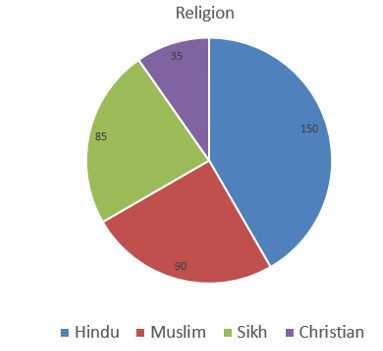Exercise 24B
OBJECTIVE QUESTIONS
Select the correct answer in each of the following:
1. A man’s monthly salary is ₹24000 and his monthly expenses on travel are ₹2500. The central angle of the sector representing travel expenses in the pie chart would be

1. 30o
2. 37 ½ o
3. 45o
4. 60o

Solution: We know that the monthly salary = ₹24000
Monthly expense on travel = ₹2500
By using the formula,
Central angle of component = ((value of the component)/Total value) × 360o
= (2500 / 24000) × 360o
= 37.5o = 37 ½ o
2. If 35% of the people residing in a locality are Sikhs then the central angle of the sector representing the Sikh community in the pie chart would be

1. 108o
2. 115o
3. 126o
4. 135o

Solution: We know that the total people residing in locality = 100 %
Sikh people residing in the same locality = 35%
By using the formula,
Central angle of component = ((value of the component)/Total value) × 360o
= (35 / 100) × 360o
= 126o

## RS Aggarwal Solutions for Class 8 Maths Chapter 24 – Pie Charts

Chapter 24- Pie Charts contains 2 exercises and the RS Aggarwal Solutions present on this page provide the solutions for the questions present in each exercise. Now, let us have a look at the concepts discussed in this Chapter.

• Calculating Central Angle for a component.
• Construction of a Pie Chart for a given data.

### Chapter Brief of RS Aggarwal Solutions for Class 8 Maths Chapter 24 – Pie Charts

RS Aggarwal Solutions for Class 8 Maths Chapter 24 – Pie Charts, are designed in such a way that they are easy to understand and solve. By regular revision, students become more clear with the concepts and can score well in their exams.

As the Chapter is about Pie Charts, here we calculate the Central angle for a component then we draw a Pie Chart based on the results obtained. In the RS Aggarwal Solutions PDF, many such exercise problems are available.